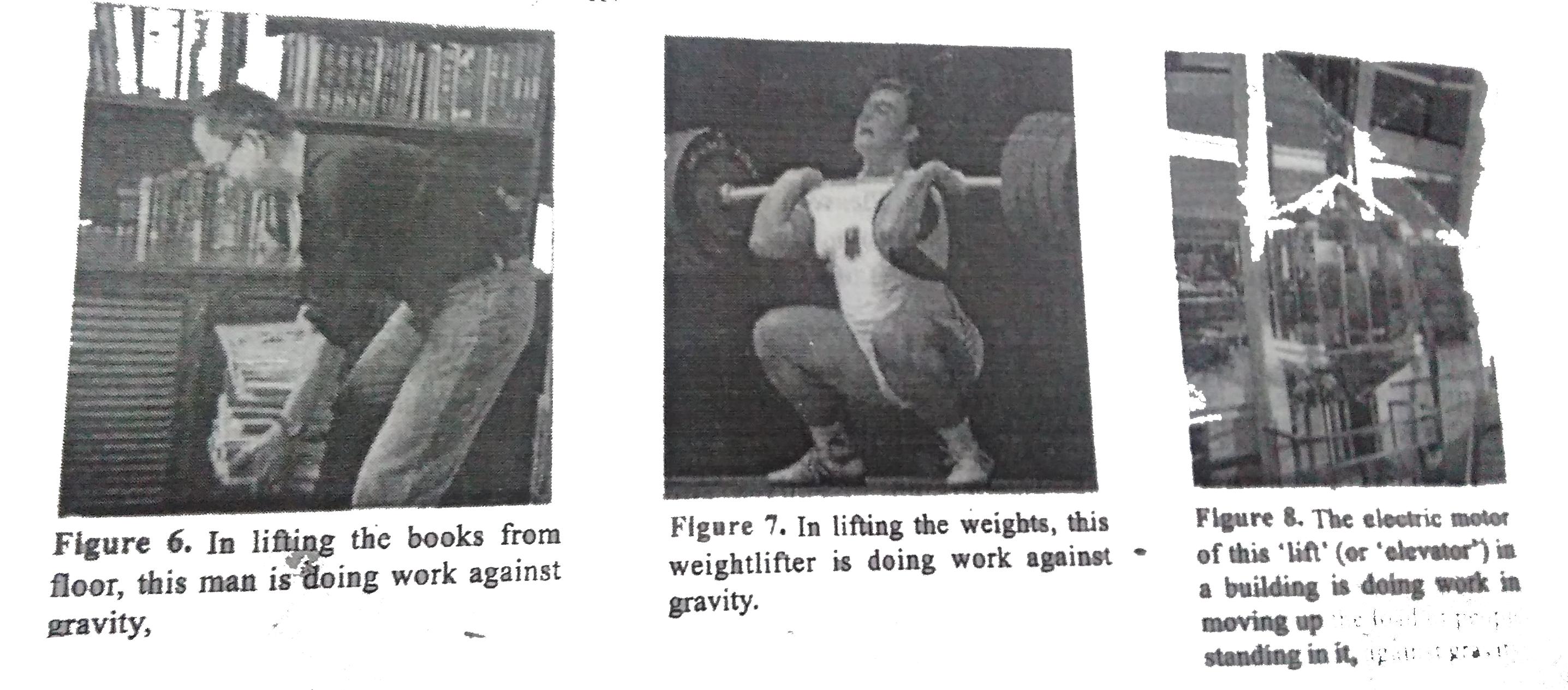Question

Updated on:26/04/2023

# Calculate the work done in lifting 200 kg of water through a vertical height of 6 meters (Assume g=10m/s2).

Text Solution

Solution
In this case work is being done against gravity in lifting water. Now, the formula for calculating the work done against gravity is :
W=m×g×h
Here, Mass of water, m = 200 kg
Acceleration due to gravity, g=10m/s2
And, Height, h = 6 m
Now putting these values in the above formula, we get :
W=200×10×6
W = 12000 J
Thus, the work done is 12000 joules.